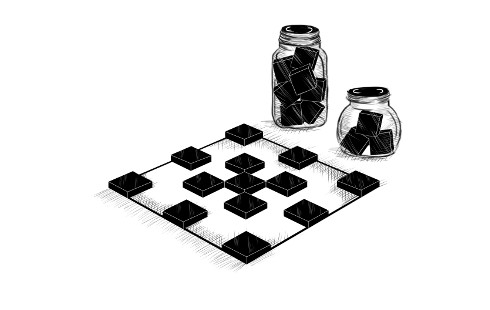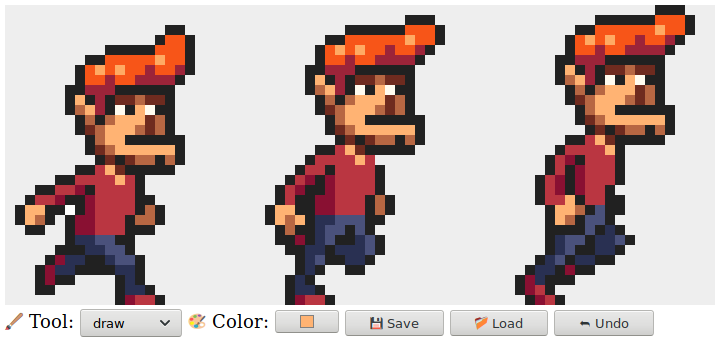# 十九、项目：像素艺术编辑器

Joan Miro## 状态

``````class Picture {
constructor(width, height, pixels) {
this.width = width;
this.height = height;
this.pixels = pixels;
}
static empty(width, height, color) {
let pixels = new Array(width * height).fill(color);
return new Picture(width, height, pixels);
}
pixel(x, y) {
return this.pixels[x + y * this.width];
}
draw(pixels) {
let copy = this.pixels.slice();
for (let {x, y, color} of pixels) {
copy[x + y * this.width] = color;
}
return new Picture(this.width, this.height, copy);
}
}
``````

`empty`方法使用我们以前没有见过的两个数组功能。 可以使用数字调用`Array`构造器来创建给定长度的空数组。 然后`fill`方法可以用于使用给定值填充数组。 这些用于创建一个数组，所有像素具有相同颜色。

``````function updateState(state, action) {
return Object.assign({}, state, action);
}
``````

## DOM 的构建

``````function elt(type, props, ...children) {
let dom = document.createElement(type);
if (props) Object.assign(dom, props);
for (let child of children) {
if (typeof child != "string") dom.appendChild(child);
else dom.appendChild(document.createTextNode(child));
}
return dom;
}
``````

``````<body>
<script>
document.body.appendChild(elt("button", {
onclick: () => console.log("click")
}, "The button"));
</script>
</body>
``````

## 画布

``````const scale = 10;

class PictureCanvas {
constructor(picture, pointerDown) {
this.dom = elt("canvas", {
onmousedown: event => this.mouse(event, pointerDown),
ontouchstart: event => this.touch(event, pointerDown)
});
drawPicture(picture, this.dom, scale);
}
setState(picture) {
if (this.picture == picture) return;
this.picture = picture;
drawPicture(this.picture, this.dom, scale);
}
}
``````

``````function drawPicture(picture, canvas, scale) {
canvas.width = picture.width * scale;
canvas.height = picture.height * scale;
let cx = canvas.getContext("2d");

for (let y = 0; y < picture.height; y++) {
for (let x = 0; x < picture.width; x++) {
cx.fillStyle = picture.pixel(x, y);
cx.fillRect(x * scale, y * scale, scale, scale);
}
}
}
``````

``````PictureCanvas.prototype.mouse = function(downEvent, onDown) {
if (downEvent.button != 0) return;
let pos = pointerPosition(downEvent, this.dom);
let onMove = onDown(pos);
if (!onMove) return;
let move = moveEvent => {
if (moveEvent.buttons == 0) {
this.dom.removeEventListener("mousemove", move);
} else {
let newPos = pointerPosition(moveEvent, this.dom);
if (newPos.x == pos.x && newPos.y == pos.y) return;
pos = newPos;
onMove(newPos);
}
};
};

function pointerPosition(pos, domNode) {
let rect = domNode.getBoundingClientRect();
return {x: Math.floor((pos.clientX - rect.left) / scale),
y: Math.floor((pos.clientY - rect.top) / scale)};
}
``````

``````PictureCanvas.prototype.touch = function(startEvent,
onDown) {
let pos = pointerPosition(startEvent.touches, this.dom);
let onMove = onDown(pos);
startEvent.preventDefault();
if (!onMove) return;
let move = moveEvent => {
let newPos = pointerPosition(moveEvent.touches,
this.dom);
if (newPos.x == pos.x && newPos.y == pos.y) return;
pos = newPos;
onMove(newPos);
};
let end = () => {
this.dom.removeEventListener("touchmove", move);
this.dom.removeEventListener("touchend", end);
};
};
``````

## 应用

``````class PixelEditor {
constructor(state, config) {
let {tools, controls, dispatch} = config;
this.state = state;

this.canvas = new PictureCanvas(state.picture, pos => {
let tool = tools[this.state.tool];
let onMove = tool(pos, this.state, dispatch);
if (onMove) return pos => onMove(pos, this.state);
});
this.controls = controls.map(
Control => new Control(state, config));
this.dom = elt("div", {}, this.canvas.dom, elt("br"),
...this.controls.reduce(
(a, c) => a.concat(" ", c.dom), []));
}
setState(state) {
this.state = state;
this.canvas.setState(state.picture);
for (let ctrl of this.controls) ctrl.setState(state);
}
}
``````

``````class ToolSelect {
constructor(state, {tools, dispatch}) {
this.select = elt("select", {
onchange: () => dispatch({tool: this.select.value})
}, ...Object.keys(tools).map(name => elt("option", {
selected: name == state.tool
}, name)));
this.dom = elt("label", null, "🖌 Tool: ", this.select);
}
setState(state) { this.select.value = state.tool; }
}
``````

``````class ColorSelect {
constructor(state, {dispatch}) {
this.input = elt("input", {
type: "color",
value: state.color,
onchange: () => dispatch({color: this.input.value})
});
this.dom = elt("label", null, "🎨 Color: ", this.input);
}
setState(state) { this.input.value = state.color; }
}
``````

## 绘图工具

``````function draw(pos, state, dispatch) {
function drawPixel({x, y}, state) {
let drawn = {x, y, color: state.color};
dispatch({picture: state.picture.draw([drawn])});
}
drawPixel(pos, state);
return drawPixel;
}
``````

``````function rectangle(start, state, dispatch) {
function drawRectangle(pos) {
let xStart = Math.min(start.x, pos.x);
let yStart = Math.min(start.y, pos.y);
let xEnd = Math.max(start.x, pos.x);
let yEnd = Math.max(start.y, pos.y);
let drawn = [];
for (let y = yStart; y <= yEnd; y++) {
for (let x = xStart; x <= xEnd; x++) {
drawn.push({x, y, color: state.color});
}
}
dispatch({picture: state.picture.draw(drawn)});
}
drawRectangle(start);
return drawRectangle;
}
````````````const around = [{dx: -1, dy: 0}, {dx: 1, dy: 0},
{dx: 0, dy: -1}, {dx: 0, dy: 1}];

function fill({x, y}, state, dispatch) {
let targetColor = state.picture.pixel(x, y);
let drawn = [{x, y, color: state.color}];
for (let done = 0; done < drawn.length; done++) {
for (let {dx, dy} of around) {
let x = drawn[done].x + dx, y = drawn[done].y + dy;
if (x >= 0 && x < state.picture.width &&
y >= 0 && y < state.picture.height &&
state.picture.pixel(x, y) == targetColor &&
!drawn.some(p => p.x == x && p.y == y)) {
drawn.push({x, y, color: state.color});
}
}
}
dispatch({picture: state.picture.draw(drawn)});
}
``````

``````function pick(pos, state, dispatch) {
dispatch({color: state.picture.pixel(pos.x, pos.y)});
}
``````

``````<div></div>
<script>
let state = {
tool: "draw",
color: "#000000",
picture: Picture.empty(60, 30, "#f0f0f0")
};
let app = new PixelEditor(state, {
tools: {draw, fill, rectangle, pick},
controls: [ToolSelect, ColorSelect],
dispatch(action) {
app.setState(state);
}
});
document.querySelector("div").appendChild(app.dom);
</script>
``````

## 保存和加载

``````class SaveButton {
constructor(state) {
this.picture = state.picture;
this.dom = elt("button", {
onclick: () => this.save()
}, "\u{1f4be} Save");
}
save() {
let canvas = elt("canvas");
drawPicture(this.picture, canvas, 1);
href: canvas.toDataURL(),
});
}
setState(state) { this.picture = state.picture; }
}
``````

`canvas`元素上的`toDataURL`方法创建一个以`data:`开头的 URL。 与`http:``https:`的 URL 不同，数据 URL 在 URL 中包含整个资源。 它们通常很长，但它们允许我们在浏览器中，创建任意图片的可用链接。

``````class LoadButton {
constructor(_, {dispatch}) {
this.dom = elt("button", {
}
setState() {}
}

let input = elt("input", {
type: "file",
});
document.body.appendChild(input);
input.click();
input.remove();
}
``````

``````function finishLoad(file, dispatch) {
if (file == null) return;
let image = elt("img", {
picture: pictureFromImage(image)
}),
});
});
}
``````

``````function pictureFromImage(image) {
let width = Math.min(100, image.width);
let height = Math.min(100, image.height);
let canvas = elt("canvas", {width, height});
let cx = canvas.getContext("2d");
cx.drawImage(image, 0, 0);
let pixels = [];
let {data} = cx.getImageData(0, 0, width, height);

function hex(n) {
}
for (let i = 0; i < data.length; i += 4) {
let [r, g, b] = data.slice(i, i + 3);
pixels.push("#" + hex(r) + hex(g) + hex(b));
}
return new Picture(width, height, pixels);
}
``````

`getImageData`返回的对象的`data`属性，是一个颜色分量的数组。 对于由参数指定的矩形中的每个像素，它包含四个值，分别表示像素颜色的红色，绿色，蓝色和 alpha 分量，数字介于 0 和 255 之间。alpha 分量表示不透明度 - 当它是零时像素是完全透明的，当它是 255 时，它是完全不透明的。出于我们的目的，我们可以忽略它。

## 撤销历史

``````function historyUpdateState(state, action) {
if (action.undo == true) {
if (state.done.length == 0) return state;
return Object.assign({}, state, {
picture: state.done,
done: state.done.slice(1),
doneAt: 0
});
} else if (action.picture &&
state.doneAt < Date.now() - 1000) {
return Object.assign({}, state, action, {
done: [state.picture, ...state.done],
doneAt: Date.now()
});
} else {
return Object.assign({}, state, action);
}
}
``````

``````class UndoButton {
constructor(state, {dispatch}) {
this.dom = elt("button", {
onclick: () => dispatch({undo: true}),
disabled: state.done.length == 0
}, "⮪ Undo");
}
setState(state) {
this.dom.disabled = state.done.length == 0;
}
}
``````

## 让我们绘图吧

``````const startState = {
tool: "draw",
color: "#000000",
picture: Picture.empty(60, 30, "#f0f0f0"),
done: [],
doneAt: 0
};

const baseTools = {draw, fill, rectangle, pick};

const baseControls = [
];

function startPixelEditor({state = startState,
tools = baseTools,
controls = baseControls}) {
let app = new PixelEditor(state, {
tools,
controls,
dispatch(action) {
app.setState(state);
}
});
return app.dom;
}
``````

``````<div></div>
<script>
document.querySelector("div")
.appendChild(startPixelEditor({}));
</script>
``````

## 练习

### 键盘绑定

``````<div></div>
<script>
// The original PixelEditor class. Extend the constructor.
class PixelEditor {
constructor(state, config) {
let {tools, controls, dispatch} = config;
this.state = state;

this.canvas = new PictureCanvas(state.picture, pos => {
let tool = tools[this.state.tool];
let onMove = tool(pos, this.state, dispatch);
if (onMove) {
return pos => onMove(pos, this.state, dispatch);
}
});
this.controls = controls.map(
Control => new Control(state, config));
this.dom = elt("div", {}, this.canvas.dom, elt("br"),
...this.controls.reduce(
(a, c) => a.concat(" ", c.dom), []));
}
setState(state) {
this.state = state;
this.canvas.setState(state.picture);
for (let ctrl of this.controls) ctrl.setState(state);
}
}

document.querySelector("div")
.appendChild(startPixelEditor({}));
</script>
``````

### 高效绘图

``````<div></div>
<script>
// Change this method
PictureCanvas.prototype.setState = function(picture) {
if (this.picture == picture) return;
this.picture = picture;
drawPicture(this.picture, this.dom, scale);
};

// You may want to use or change this as well
function drawPicture(picture, canvas, scale) {
canvas.width = picture.width * scale;
canvas.height = picture.height * scale;
let cx = canvas.getContext("2d");

for (let y = 0; y < picture.height; y++) {
for (let x = 0; x < picture.width; x++) {
cx.fillStyle = picture.pixel(x, y);
cx.fillRect(x * scale, y * scale, scale, scale);
}
}
}

document.querySelector("div")
.appendChild(startPixelEditor({}));
</script>
``````

### 圆

``````<div></div>
<script>
function circle(pos, state, dispatch) {
}

let dom = startPixelEditor({
tools: Object.assign({}, baseTools, {circle})
});
document.querySelector("div").appendChild(dom);
</script>
``````

### 合适的直线``````<div></div>
<script>
// The old draw tool. Rewrite this.
function draw(pos, state, dispatch) {
function drawPixel({x, y}, state) {
let drawn = {x, y, color: state.color};
dispatch({picture: state.picture.draw([drawn])});
}
drawPixel(pos, state);
return drawPixel;
}

function line(pos, state, dispatch) {